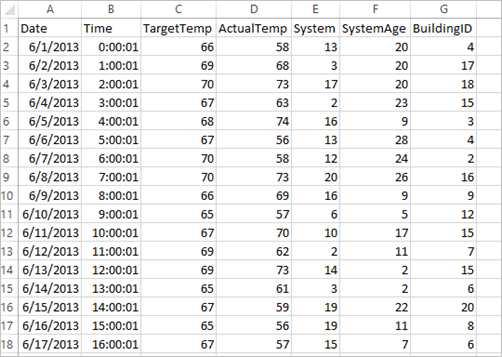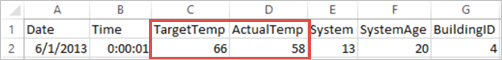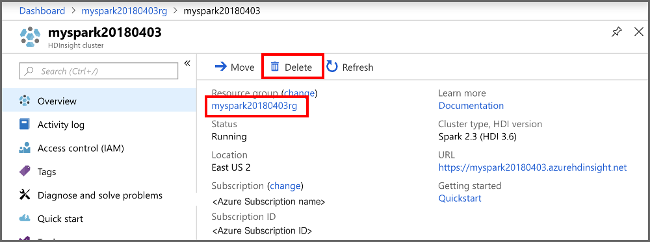# 教程：在 Azure HDInsight 中生成 Apache Spark 机器学习应用程序

MLlib 是 Spark 的自适应机器学习库，由常见的学习算法和实用工具组成。 （分类、回归、聚类、协作筛选和维数约简， 以及底层优化基元。）

• 开发 Apache Spark 机器学习应用程序

## 了解数据集## 使用 Spark MLlib 开发 Spark 机器学习应用程序

1. 使用 PySpark 内核创建 Jupyter Notebook。 有关说明，请参阅创建 Jupyter Notebook

2. 导入此方案所需的类型。 将以下代码段粘贴到空白单元格中，然后按 Shift+Enter

``````from pyspark.ml import Pipeline
from pyspark.ml.classification import LogisticRegression
from pyspark.ml.feature import HashingTF, Tokenizer
from pyspark.sql import Row

import os
import sys
from pyspark.sql.types import *

from pyspark.mllib.classification import LogisticRegressionWithSGD
from pyspark.mllib.regression import LabeledPoint
from numpy import array
``````
3. 加载数据 (hvac.csv)，分析数据，并使用它来训练模型。

``````# Define a type called LabelDocument
LabeledDocument = Row("BuildingID", "SystemInfo", "label")

# Define a function that parses the raw CSV file and returns an object of type LabeledDocument
def parseDocument(line):
values = [str(x) for x in line.split(',')]
if (values > values):
hot = 1.0
else:
hot = 0.0

textValue = str(values) + " " + str(values)

return LabeledDocument((values), textValue, hot)

# Load the raw HVAC.csv file, parse it using the function
data = sc.textFile("/HdiSamples/HdiSamples/SensorSampleData/hvac/HVAC.csv")

documents = data.filter(lambda s: "Date" not in s).map(parseDocument)
training = documents.toDF()
``````

在此代码片段中，定义用于比较实际温度与目标温度的函数。 如果实际温度较高，则表示建筑物处于高温状态，以值 1.0 表示。 否则建筑物处于低温状态，用值 0.0 表示。

4. 设置包括三个阶段的 Spark 机器学习管道：tokenizer、hashingTF 和 lr。

``````tokenizer = Tokenizer(inputCol="SystemInfo", outputCol="words")
hashingTF = HashingTF(inputCol=tokenizer.getOutputCol(), outputCol="features")
lr = LogisticRegression(maxIter=10, regParam=0.01)
pipeline = Pipeline(stages=[tokenizer, hashingTF, lr])
``````

有关管道及其工作原理的详细信息，请参阅 Apache Spark 机器学习管道

5. 将管道拟合到培训文档中。

``````model = pipeline.fit(training)
``````
6. 验证训练文档以根据应用程序的进度创建检查点。

``````training.show()
``````

输出类似于：

``````+----------+----------+-----+
|BuildingID|SystemInfo|label|
+----------+----------+-----+
|         4|     13 20|  0.0|
|        17|      3 20|  0.0|
|        18|     17 20|  1.0|
|        15|      2 23|  0.0|
|         3|      16 9|  1.0|
|         4|     13 28|  0.0|
|         2|     12 24|  0.0|
|        16|     20 26|  1.0|
|         9|      16 9|  1.0|
|        12|       6 5|  0.0|
|        15|     10 17|  1.0|
|         7|      2 11|  0.0|
|        15|      14 2|  1.0|
|         6|       3 2|  0.0|
|        20|     19 22|  0.0|
|         8|     19 11|  0.0|
|         6|      15 7|  0.0|
|        13|      12 5|  0.0|
|         4|      8 22|  0.0|
|         7|      17 5|  0.0|
+----------+----------+-----+
``````

将输出与原始 CSV 文件进行比较。 例如，CSV 文件中第一行包含此数据：请注意，实际温度比目标温度低的情况表示建筑物处于低温状态。 第一行中的标签值为 0.0，表示建筑物温度不高 。

7. 准备要对其运行已训练模型的数据集。 为此，需要传递系统 ID 和系统年数（在训练输出中以 SystemInfo 表示）。 模型将预测具有该系统 ID 和系统年数的建筑物温度是更高（由 1.0 表示）还是更低（由 0.0 表示）。

``````# SystemInfo here is a combination of system ID followed by system age
Document = Row("id", "SystemInfo")
test = sc.parallelize([(1L, "20 25"),
(2L, "4 15"),
(3L, "16 9"),
(4L, "9 22"),
(5L, "17 10"),
(6L, "7 22")]) \
.map(lambda x: Document(*x)).toDF()
``````
8. 最后，对测试数据进行预测。

``````# Make predictions on test documents and print columns of interest
prediction = model.transform(test)
selected = prediction.select("SystemInfo", "prediction", "probability")
for row in selected.collect():
print row
``````

输出类似于：

``````Row(SystemInfo=u'20 25', prediction=1.0, probability=DenseVector([0.4999, 0.5001]))
Row(SystemInfo=u'4 15', prediction=0.0, probability=DenseVector([0.5016, 0.4984]))
Row(SystemInfo=u'16 9', prediction=1.0, probability=DenseVector([0.4785, 0.5215]))
Row(SystemInfo=u'9 22', prediction=1.0, probability=DenseVector([0.4549, 0.5451]))
Row(SystemInfo=u'17 10', prediction=1.0, probability=DenseVector([0.4925, 0.5075]))
Row(SystemInfo=u'7 22', prediction=0.0, probability=DenseVector([0.5015, 0.4985]))
``````

观察预测结果中的第一行。 对于 ID 为 20 且系统年数为 25 年的 HVAC 系统，建筑物温度很高（预测结果=1.0）。 DenseVector (0.49999) 的第一个值对应于预测 0.0，第二个值 (0.5001) 对应于预测 1.0。 在输出中，即使第二个值只稍高一点，模型也仍旧显示 prediction=1.0

9. 关闭笔记本以释放资源。 为此，请在 Notebook 的“文件”菜单中选择“关闭并停止” 。 此操作会关闭 Notebook。

## 将 Anaconda scikit-learn 库用于 Spark 机器学习

HDInsight 中的 Apache Spark 群集包含 Anaconda 库。 它还包括适用于机器学习的 scikit-learn 库。 该库还包含用于直接从 Jupyter 笔记本生成示例应用程序的各种数据集。 有关使用 scikit-learn 库的示例，请参阅 https://scikit-learn.org/stable/auto_examples/index.html

## 清理资源

1. 登录 Azure 门户

2. 在顶部的“搜索”框中，键入 HDInsight

3. 选择“服务”下的“HDInsight 群集” 。

4. 在显示的 HDInsight 群集列表中，选择为本教程创建的群集旁边的“...”。

5. 选择“删除”。 请选择“是”。